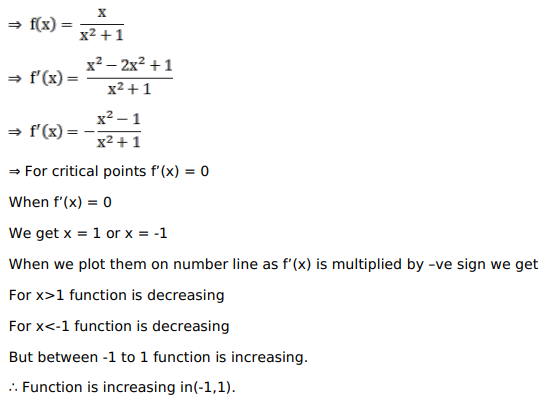# Solve this following

Question:

Mark $(\sqrt{)}$ against the correct answer in the following:

$f(x)=\frac{x}{\left(x^{2}-1\right)}$ is increasing in

A. $(-1,1)$

B. $(-1, \infty)$

C. $(-\infty,-1) \cup(1, \infty)$

D. none of these

Solution: